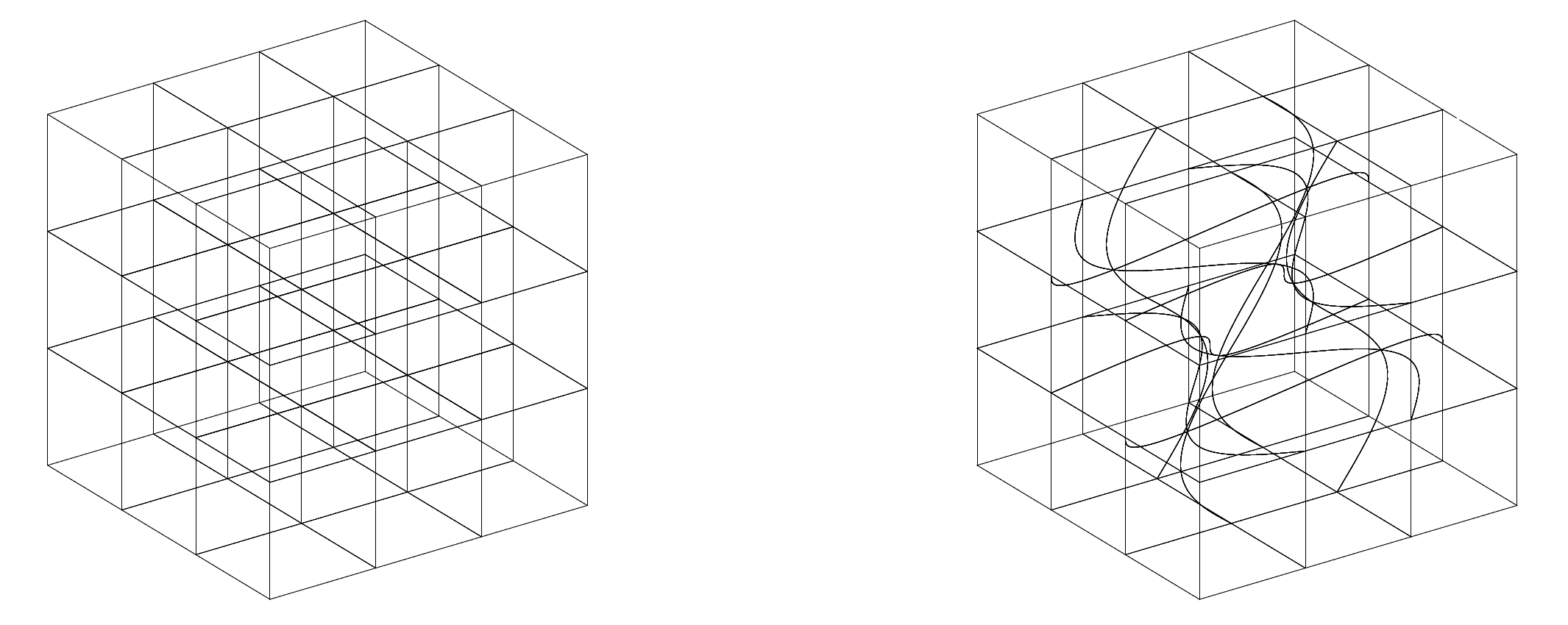# crazy_mesh.py¶

In this script, we define a mesh in a unit cube,The mesh is ofelements,The mappingis given aswhereis a linear mapping,, i.e.,andis a mapping,whereis a deformation factor. When,is also a linear mapping. Thus we have a uniform orthogonal mesh. When, the mesh is curvilinear. Two examples (left:, right:) of this mesh forare shown below.⭕ To access the source code, click on the [source] button at the right side or click on [crazy_mesh.py]. Dependence may exist. In case of error, check import and install required packages or download required scripts. © mathischeap.com

class crazy_mesh.CrazyMesh(c, K)[source]

The crazy mesh.

Parameters:
• c (float) – The deformation factor,.

• K (int) – The crazy mesh is ofelements.

Example:

>>> cm = CrazyMesh(0.1, 2)
>>> e0 = cm.CT_of_element_number(0)
>>> e000 = cm.CT_of_element_index(0, 0, 0)
>>> e0 is e000
True
>>> e7 = cm.CT_of_element_number(7)
>>> e111 = cm.CT_of_element_index(1, 1, 1)
>>> e7 is e111
True
>>> e000
<coordinate_transformation.CoordinateTransformation object at...
>>> e7
<coordinate_transformation.CoordinateTransformation object at...

CT_of_element_index(i, j, k)[source]

Return a CoordinateTransformation instance for element.

Note that Python index starts from. So for a CrazyMesh ofelements, its indices,.

Parameters:
• i (int) – Element index i.

• j (int) – Element index j.

• k (int) – Element index k.

Returns:

A CoordinateTransformation instance.

CT_of_element_number(m)[source]

Return a CoordinateTransformation instance for element.

Note that Python index starts from. So for a CrazyMesh ofelements,.

Parameters:

m (int) – Element No. m.

Returns:

A CoordinateTransformation instance.

class crazy_mesh.CrazyMeshGlobalBoundaryDOFs(K, N)[source]

We find the global numbering of the dofs on each boundary of the crazy mesh.

Parameters:
• K (int) – The crazy mesh is ofelements.

• N (int) – The degree. of the to be used mimetic polynomial basis functions.

Example:

>>> K = 2
>>> N = 1
>>> B_DOFs = CrazyMeshGlobalBoundaryDOFs(K, N)
>>> FB_dofs = B_DOFs.FP
>>> FB_dofs['x_minus'] # x=0 face
array([0, 3, 6, 9])
>>> FB_dofs['x_plus'] # x=1 face
array([ 2,  5,  8, 11])
>>> FB_dofs['y_minus'] # y=0 face
array([12, 13, 18, 19])
>>> FB_dofs['y_plus'] # y=1 face
array([16, 17, 22, 23])
>>> FB_dofs['z_minus'] # z=0 face
array([24, 25, 26, 27])
>>> FB_dofs['z_plus'] # z=1 face
array([32, 33, 34, 35])

property EP

Find the dofs of an element inwhich are on boundary of the crazy mesh ofelements.

property FP

Find the dofs of an element inwhich are on boundary of the crazy mesh ofelements.

Returns:

A dict whose keys are ‘x_minus’, ‘x_plus’, ‘y_minus’, ‘y_plus’, ‘z_minus’, ‘z_plus’, and whose values are the global numbering of the dofs on the boundaries indicated by the keys.

property NP

Find the dofs of an element inwhich are on boundary of the crazy mesh ofelements.

class crazy_mesh.CrazyMeshGlobalNumbering(K, N)[source]

A wrapper of global numberings for discrete variables in the crazy mesh.

Parameters:
• K (int) – The crazy mesh is ofelements.

• N (int) – The degree. of the to be used mimetic polynomial basis functions.

Example:

>>> K = 2
>>> N = 1
>>> GM = CrazyMeshGlobalNumbering(K, N)
>>> GM.FP
array([[ 0,  1, 12, 14, 24, 28],
[ 1,  2, 13, 15, 25, 29],
[ 3,  4, 14, 16, 26, 30],
[ 4,  5, 15, 17, 27, 31],
[ 6,  7, 18, 20, 28, 32],
[ 7,  8, 19, 21, 29, 33],
[ 9, 10, 20, 22, 30, 34],
[10, 11, 21, 23, 31, 35]])
>>> GM.VP
array([,
,
,
,
,
,
,
])

property EP

Generate a global numbering for the dofs of an element inon a crazy mesh ofelements.

property FP

Generate a global numbering for the dofs of an element inon a crazy mesh ofelements.

property NP

Generate a global numbering for the dofs of an element inon a crazy mesh ofelements.

property VP

Generate a global numbering for the dofs of an element inon a crazy mesh ofelements.

↩️ Back to Ph.D. thesis complements (ptc).